## ECE 476 Final Project: Voice Compression using ADPCM algorithm

by Rishi Sinha (rks33) and William Chung (wc227)
Wednesday 4:30pm lab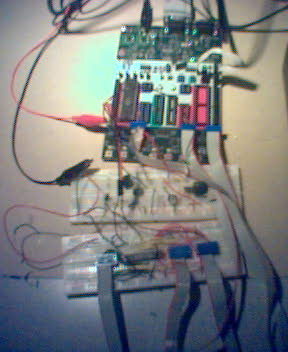### Introduction:

Digital voice recording using 2- and 4-bit ADPCM!

An 8 or 16 second digital voice recorder was implemented which utilized the ADPCM algorithm for lossy speech compression. We stored our speech samples on a 32 KB SRAM. The Atmel Mega32 microcontroller was used to run the ADPCM algorithm, to interface with the SRAM, to output sound to the B/W TV, and to sample the output from a microphone circuit.

### High Level Design:

This section provides the rationale for our project.

2.1 Where did our project idea come from?

We thoroughly enjoyed doing lab 2 (the DTMF/speech synthesis lab). We thought the speech sounded ok, and thought there might be a better way to do (lossy) speech compression. The speech synthesis page indicated that ADPCM would be a good algorithm to improve speech quality. The page also indicated that it would be a good idea to implement for a final project, so we thought we try that out. To make things more interesting, we decided to integrate the algorithm into a simple digital voice recorder.

2.2 Logical Structure

The following is a top level diagram of our digital voice recorder.The ADPCM encoder works as follows. Each input speech sample is differenced with a reconstructed estimate of the sample. This differenced sample is then quantized and assigned to a valid pulse code. The motivation is that the differenced signal has a smaller amplitude swing compared with the original signal, and thus, the quantizer levels can be spaced closer together to reduce quantizer noise. This also indicates that the algorithm will work better as the sampling frequency increases.

The ADPCM decoder works as follows. Each input pulse code maps to a quantizer level. This level is then added to a running sum to generate an approximation to the original speech sample.

What makes the algorithm adaptive is that the quantizer levels change with each speech sample. When the difference signal is large, the quantizer levels are large. When the difference signal is small, the quantizer levels are small. Thus, the design of the algorithm entails specifying how the quantizer should respond to the changing difference signal.

How should one resize the quantizer levels? A simple method would be to double the size of the quantizer should the algorithm produces a difference signal that clips the quantizer (-7 or +7 for 4-bit ADPCM). In fact, this is what is done in practice. How to resize the quantizer levels when the pulse code doesn’t clip isn’t as apparent, but the above suggests an exponential scheme to increase and decrease the size of the quantizer levels.

What makes the ADPCM algorithm attractive is that both the encoder and decoder do not require precision multiply and adds. Simple logical shifts and adds suffice.

2.4 Background Math

Both  and  use a geometric scheme to adjust the quantizer. The size of the quantizer levels are varied geometrically according to the difference signal. Let “stepsize” denote the size of the largest quantizer level. The geometric stepsize model is completely characterized by four parameters:

• starting value, s
• ending value, e
• base, b
• function f: difference signal → change in stepsize.

The starting value, s, specifies the minimum value of stepsize. The ending value, e, specifies the maximum value of stepsize. The base refers to b in b^n. The function f specifies how the quantizer levels should resize itself in response to the difference signal.

Both  and  (4-bit ADPCM) use the same base (b = 1.1) and the same function f. The table below specifies the function f:

 Absolute Value of Difference Signal Change in Stepsize 0 /1.1 1 /1.1 2 /1.1 3 /1.1 4 *1.1^2 5 *1.1^4 6 *1.1^6 7 *1.1^8

However, they differ in s and e due to a slightly different implementation of the quantizer and due to  having a 16-bit wide DAC and  having a 12-bit wide DAC.

The exponential stepsize scheme is implemented as a two-step lookup table to facilitate fast computation. First the parameters s, e, and b are used to generate a geometric look up table (see tabledesign2.m and tabledesign4.m in the Appendix). s is the first value in the table and e is the last value in the table. Next, the function f is implemented by shifting the index of the geometric lookup table (see the variable index in encode2.m and encode4.m ). For example, multiplying the current stepsize by 1.1 6 is the same as moving the index of the geometric table 6 spaces to the right.

2.5 Implementation

For our project, we implemented both 4-bit ADPCM and 2-bit ADPCM. Since all speech signals are ADC’ed to 8 bits, the 4-bit version offers a 50% storage savings while the 2-bit version offers a 75% storage savings. Furthermore, all speech signals are assumed to be sampled at 7812 Hz. Thus, each second of sound consists of 7812 samples. 4-bit ADPCM uses 4-bits per sample which means 1 second of sound will take up approximately 32,000 bits or 4,000 bytes. Likewise, 2-bit ADPCM will require approximately 2,000 bytes to encode 1 second of sound. Since our external SRAM has 32 KB of flash memory, it can store a bit more than 8 second of sound with 4-bit ADPCM and a bit more than 16.5 seconds of sound with 2-bit ADPCM.

Both the 2-bit and 4-bit algorithms were first implemented in Matlab (see appendix) to ensure correctness. Next, we adjusted the parameters to find the ones that yield an output sound signal that subjectively sound the best to us (see next section). Then, we compressed the output sound signal into a usable form for the MCU. To test our algorithm, we used the 8-bit, 8 kHz “digits8.wav” file from lab 2 as the input. We assume that this file is the full fidelity speech signal that all subsequent output signals will be compared to.

2.6 Parameters

Our 4-bit ADPCM implementation uses the following parameters:

 Base b 2^1/8 (1.09) Startvalue s 7 Endvalue e 127 (2^ 7 – 1) Function f same as  and 

The base b and the function f were chosen because  claims that these values optimize the algorithm. The fact that  uses the same parameters makes it likelier that these values actually do optimize the algorithm. The startvalue s was chosen to quantize the minimum level possible. The endvalue were chosen to reflect the fact that the ATMEGA32 DAC is only 8 bits wide.

The parameter values yield an SNR of 20.1272 for our test signal “digits.wav”. The 4 bit version of the algorithm is very good, but it is also clearly worse that the original 8-bit version. The 4-bit version is also vastly superior to the 2-bit version as we shall see.

Our 2-bit ADPCM implementation uses the following parameters:

Base b 2^1/8 (1.09)
Startvalue s 1
Endvalue e 127 (2^ 7 – 1)
Function f
 Absolute value of the difference Change in Stepsize 0 1 /1.1 *1.1^4

These values gave us a SNR of 12.1777 dB for our test signal, which is the highest value we obtained from a trial and error search for the best parameters. Our search criterion was SNR. We should note that our search for the best parameters wasn’t that exhaustive. Even with these parameters, the sound is very scratchy. We think that the DCPM version (lab 2) of “AllDigits” is better than our ADPCM version of “AllDigits.” The reader is invited to listen to the wave files we generated from both versions to decide for herself.

The ADPCM algorithm described in  has the following “feature.” When the input is a constant dc, the algorithm will reconstruct that as a triangle wave. This feature helps improve the speech when the input is varying rapidly, but also adds a bit of a high frequency (4 kHz) squeal. Luckily, when the input is dc, the differenced signal is 0, which means the algorithm will automatically adjust the stepsize to the smallest possible value. The power out of this triangle wave is very small.

While we were testing our system, we also discovered that running timer0 is PWM mode at 7812 Hz produces a 7812 Hz squeal on OC0. The power of this noise is much higher than the power of ADPCM triangle noise.

Rather than come up with software approaches (digital filtering, algorithm changes, etc.) to remove these two noise signals, we decided to use hardware filtering instead. We moved the cutoff frequency of the Chebyshev filter closer to dc and added another RC low pass filter at the output of the Chebyshev filter. The additional pole provided by the RC filter attenuates the noise by another factor of 3 dB.

Another way the PWM noise can be completely suppressed is to build our own DAC (instead of using OC0) which would consist of a latch connected to a resistor network. We didn’t do this because all our ports were used up (in memory access and AD/DA conversion). However, it is possible to address the SRAM using only 1 port (which would allow us to free a port for a DAC). We chose not to do so because of the timing constraints on us to complete the project.

3 Software/Hardware Design

3.1 Program Details

Our code was written using DCPMnums.c that Professor Land provided in lab 2, as a template.

Both the ADPCM encoder and decoder are run by timer0 in PWM mode to ensure regular interrupts and to free OC0 for DA conversion. A state variable called buttonPressed determines whether the encoding algorithm is run, the decoding algorithm is run, or if timer0 should turn itself off. Events timed by timer2 causes the state variable buttonPressed to change state.

There are two events that timer2 is constantly monitoring. Both events are button pushes on the STK500. When button0 is pressed, buttonPressed is set to RECORD mode. In this mode, The ADC converts a sample from the microphone. This sample is processed by the encoding algorithm, and then stored in the SRAM. All of this is timed by timer0 is PWM mode. When button1 is pressed, buttonPressed is set to PLAYBACK mode. In this mode, the microcontroller takes a sample from the SRAM, decodes it, and writes it to OC0 (our DAC). Again, all of this is timed by timer0 in PWM mode. Both RECORD mode and PLAYBACK mode runs for a fixed amount of time. After this fixed amount of time elapses, buttonPressed goes back to STANDBY mode.

3.2 RAM Interface

We used the Toshiba SRAM TC5532P as our memory element. It has a fast (for our purposes) read/write access time of 35 ns. The chip contains 11 address pins and 8 I/O bits. Additionally, it contains a CE pin (chip enable), a WE pin (write enable), and an OE pin (output enable). See the figure below.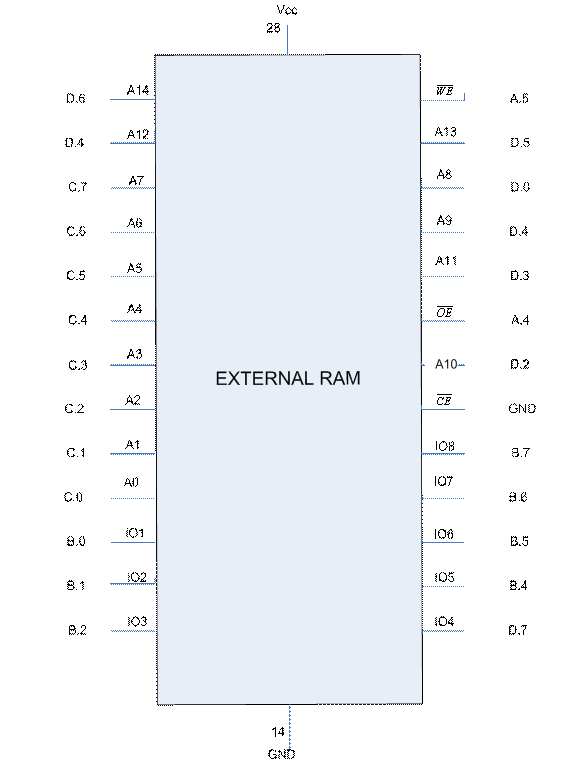In our interface, we routed PORTC and PORTD (0-6) to the address bits of the RAM. PORTB (except B.3 because it is the output of the mcu DAC) was connected to the IO pins. D.7 took the place of B.3. A.5 and A.4 were connected to WE and OE.

Reading and writing to the RAM is very simple. To read, simply set the address bits with PORTD and PORTC on one cycle, and set OE low on the next. On the third cycle, read the bits off of PINB and PIND.7. To write, again, set the address bits with PORTD and PORTC on one cycle, and set OE high on the next. On the third cycle, set WE low. On the fourth cycle, write data to PORTB and PORTD.7. On the fifth cycle, set WE high to protect data integrity.

3.3 Low Pass Filter

There are lots of high frequency components present in the signal coming out of the DAC of the MCU due to the presence of various harmonics of the fundamental frequency. So a low pass filter having a cutoff frequency of about 3.3 kHz was designed since the bandwidth of human voice is about 4 kHz. Since the cutoff frequency is small the inductors required for the design of passive filters would be enormous and they were not available in the lab. So we designed an Active filter requiring one OpAmp. First we tried an active second order Butterworth filter but this did not solve our purpose since it is a flat filter and the output takes a greater frequency range to die down completely after the cutoff frequency. In other words, it does not have skirts as sharp as the chebyshev filter. Chebyshev filter has some pass band ripple but it was considered acceptable for our purpose since the ripple amplitude is too small to affect our output voice. So an active 2 nd order chebyshev filter was designed having a 3 dB pass band ripple was designed which had the cutoff frequency of 3.3 kHz. This filter had a gain of unity. The 2 nd order chebyshev filter was followed by a first order Butterworth low pass filter having a cutoff frequency of 2.6 kHz since when we used only the 2 nd order Chebyshev filter there was a constant ringing sound in the output at a frequency of 7.8 kHz. Although the filter was designed to have a cutoff frequency of 3.3 kHz, the peak to peak value of the signal at the frequency of 7.8 kHz was not removed completely. So we designed a 1 pole Butterworth filter following the chebyshev filter to get the proper attenuation at 7.8 kHz.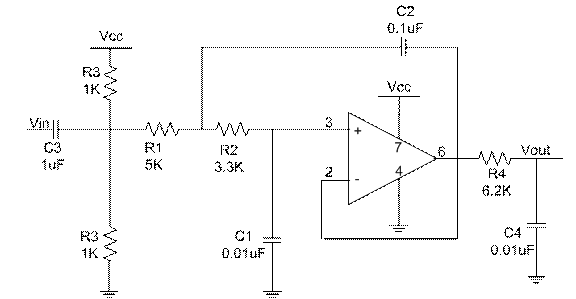2 pole Chebyshev Filter followed by a single pole Butterworth Low pass filter

The filter consists of an Active Device (opamp) LMC7111 along with a combination of resistors and capacitors to provide the performance which is equivalent to LRC circuit at low frequency. The filter takes the input from the output of DAC of the MCU and the output of the filter serves as the input to a speaker (in this case a TV) so that we can listen to the voice. The opamp requires a negative supply. So in order to overcome that difficulty we placed a bias (offset) voltage of 2.5V by placing a voltage divider and a capacitor in front of the input to filter. The capacitor blocks any DC component of the signal from the MCU to enter the filter. So it does not disturb the bias voltage which is set by the voltage divider following the capacitor before the filter input. The offset resistors R3 should not be much greater than R1 and R2. Therefore a value of 2k was picked for R3.

The transfer function of the low pass filter is equal to

A(s) = 1/ (1+ wc*C1*(R1+R2)*s + wc^2*R1*R2*C1*C2*s^2)

Comparing this with standard transfer function we get A0= 1, a1= wc*C1*(R1+R2)*s, b1= wc^2*R1*R2*C1*C2.

So we pick the value of C1= 0.01uF. C2>=C1*4*b1/a1^2. So we get C2>=.07uF. So we decided C2= 0.1uF. Calculating R1 and R2 we get R1= 620 ohms and R2= 3.3K ohms.But now R1 in parallel with 2 R3's becomes very small. So a higher value than calculated was put for R1 to get less ringing.

The component values of R4 and C4 were selected using the 2*Π*fc= 1/(R4*C4). We picked an available value of C which was 0.01 uf and R4 was found out to be 6.2k for a cutoff frequency of 2.6 kHz.

3.4 Microphone Circuit

We are getting the audio signal input from the Microphone. But the magnitude of the signal is too small and if left alone the noise present in the drive will override the audio signal. So in order to increase the SNR at the audio input to the Microcontroller we pass the audio signal through an audio preamplifier. The gain of the preamplifier depends on values of R4 and R5. Our amplifier had a gain of about a 100. We tried to increase the gain beyond this but after a certain gain the amplifier started to become saturated meaning that the output was not linearly proportional to input signal. The output sound was good enough to be audible in this case.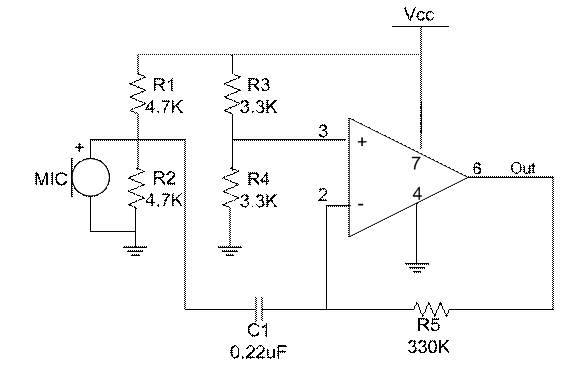Preamplifier

4 Results

Our attempt to suppress the high frequency noise signals caused by the algorithm and PWM mode went well. We removed a lot of the power, but one is still able to hear the high pitched squeal. The extra RC filter attached to the output of the Cheybyshev filter did wonders to attenuate the noise. However, we also noticed that it “blurred” the speech as well. One thing we might try to do is to implement another 2 pole Chebyshev filter for a 4-pole cascaded system.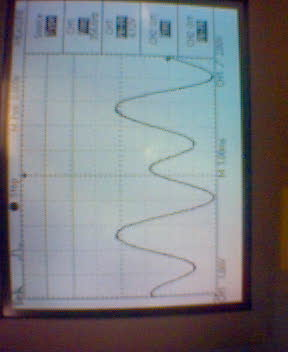Audio Input to Microcontroller through the Microphone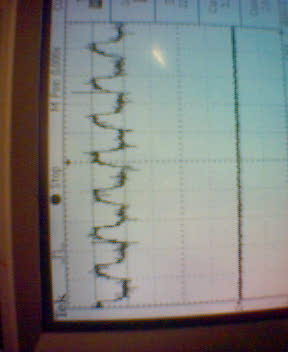Noisy PWM Output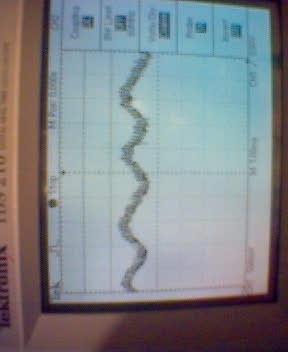Audio Output

4.1 Safety

Having dealt with many mal-functioning STK500s, we made sure to protect the one we were using. When wiring or rewiring are done, we made sure the STK500 is turned off.

4.2 Usability

Our device is very user-friendly. Pressing button0 on the STK500 will initiate a record cycle. After the record cycle, pressing button1 on the STK500 will initiate a playback cycle. As far as we know, everything is timed perfectly. The only thing tricky about our system is the following. In order to flash the mcu with our code, we must first disconnect the SRAM from the power rail. Afterwards, we can reconnect the SRAM to the power rail and our device is good to go.

5 Conclusions

Overall, the system worked. We were able to speak into the microphone and the system reproduced the speech intelligibly. The voice quality of 2-bit ADPCM isn’t very good at all, but in a constrained memory environment, it isn’t that bad of a choice. The voice quality of 4-bit ADPCM is far superior to its 2-bit cousin. The only “problem” is that we could still hear that annoying high-pitched, PWM squeal, however faint it may be.

5.1 What might we do to improve our project?

We tried modifying the algorithm to reduce distortion. One thing we tried was to implement an improved predictor. The basic algorithm estimates the current sample with the last sample (x[n] ~= x[n-1]). This is known as a 0 th order predictor (constant polynomial). We thought sound quality can be improved with a 1 st order predictor (linear polynomial) since the difference signal (or you can think of this as the error signal between the actual sample and the predicted sample) will be of smaller amplitude.

The first order predictor is a linearization about the previous sample:

x(t+dt) ~= x(t) + dx/dt * dt

which reduces to the following in discrete form:

x[n+1] ~= x[n] + (x[n] – x[n-1]) = 2x[n] – x[n-1]

However, when we tried to implement the 1st order predictor for 2-bit ADPCM, the sound quality was actually worse. We may have implemented it incorrectly. However, the 1 st order predictor did improve the sound quality for 4-bit ADPCM.

We can also try higher order predictors, but the law of diminishing returns will quickly come into effect. We can also try a statistical predictor like a Wiener filter, but that seems to be a whole new project (Linear Prediction).

5.2 Intellectual Property Considerations

We would like to mention again that our code is written using Professor Land’s DPCMnums.c as a template. The ADPCM algorithm itself was implemented as specified in  and  with slight modifications.

5.3 Ethical Considerations

As far as we know, our project is safe. Our low pass filter was designed to not load the mcu and to have enough output impedance to drive the speakers of the B/W TV. No boards or TVs were harmed as a result of our tests (we did have problems with many STK500s, but our circuit did not cause these problems).

We have not misled anyone on the performance of our device. Once again, the 2-bit ADPCM algorithm delivers bad, but intelligible speech. The 4-bit ADPCM algorithm delivers better performance. There is also a high frequency squeal during playback as a result of the mcu PWM mode. We have yet to figure out a software approach to minimize this squeal.

We believe that we have given credit to all parties that we took inspiration from. See section 5.2. We should also state that we borrowed an op-amp from the Flex lab for this project with the intention of returning said op-amp after the demo on 2006-May-3.

We did not partake in any actions that would be deemed harmful to any other group. We did not touch any other group’s project. We have, however, asked another group for permission to use one of their LMC711 op-amps during our demo. After the demo, we will return the op-amp to that group.

Acknowledgements

We would like to thank all the TAs especially Jason Chiang and Gus Lott and Professor Bruce Land for having the patience to help us to get our project done.There were times when we did not order certain parts but luckily Prof. Bruce had it in reserve. Had it not been there we would not been able to complete the project .

References

Appendices

Appendix A

Part List

 S. No. Description Quantity Price 1 STK500 1 \$15 2 Mega32 1 \$ 8 3 Breadboard 2 \$12 4 TV 1 \$ 5 5 RAM 1 \$ 4 6 Microphone 1 \$ 1 7 Power supply 2 \$ 5 8 Resistance Various Free from lab 9 Capacitors Various Free from lab

Total \$50

-------------------------------------------------------------------------------------------------------------------------------

Appendix B

This section describes the allocation of tasks.

```Software/Program related – William
Hardware related – Rishi
Software/Hardware Integration – William and Rishi
Writing Report – William and Rishi
Debugging Hardware and Software Problems – William and Rishi ```

-------------------------------------------------------------------------------------------------------------------------------

Appendix C

The following is our Matlab test code for 2-bit ADPCM. The 4-bit version of the code is analogous.

encode2.m

```% ECE 476 Final Project

clear

% Store speech signal in array x
[x fs numbits]= wavread('digits8'); % fs = 8, numbits = 8
x = x*128;
len = length(x);

% Generate the step size lookup tables
% hardcode it from the paper...
index = [-1 4];
currentIndex = 2;
tabledesign2;

% Simple 2-bit ADPCM algorithm-------------------------------------
sign_bit = 2;

ss = zeros(1,len);
ss(1) = table2(1);
z = zeros(1,len);
code = zeros(1,len);
neg = 0;

for n = 2:len
d(n) = x(n) - z(n-1);

% encoder
% compute code(n) from
if (d(n) < 0)
neg = 1;
code(n) = code(n) + sign_bit;
d(n) = -d(n);
else
neg = 0;
end

if (d(n) >= ss(n-1))
code(n) = code(n) + 1;
end

% decode the encoded sample
% calculate the quantized difference from code(n)
if (neg)
temp = code(n) - sign_bit;

else
temp = code(n);
end

temp2 = (temp+.5)*ss(n-1);
if (neg)
temp2 = -temp2;
end
z(n) = z(n-1) + temp2;
if (z(n) > 127)
z(n) = 127;
elseif (z(n) < -127)
z(n) = -127;
end

% compute the new step size
temp = temp + 1;
currentIndex = currentIndex + index(temp);
if (currentIndex < 1)
currentIndex = 1;

elseif (currentIndex > numSteps)
currentIndex = numSteps;
end

ss(n) = table2(currentIndex);
end

x = x/128; % put it back in the interval (-1,1)
-------------------------------------------------------------------------------------------------------------------------------
decode2.m
% APDCM decoder
y = zeros(1,len);
ss2 = zeros(1,len);
ss2(1) = startval;

for n = 2:len

% decode the encoded sample
% calculate the quantized difference from code(n)
neg = code(n) >= sign_bit;
if (neg)
temp = code(n) - sign_bit;
else
temp = code(n);
end
temp2 = (temp+.5)*ss2(n-1);
if (neg)
temp2 = -temp2;
end

y(n) = y(n-1) + temp2;
if (y(n) > 127)
y(n) = 127;

elseif (y(n) < -127)
y(n) = -127;
end

% compute the new step size

temp = temp + 1;
currentIndex = currentIndex + index(temp);
if (currentIndex < 1)
currentIndex = 1;
elseif (currentIndex > numSteps)
currentIndex = numSteps;
end

ss2(n) = table2(currentIndex);
end

y = y/128;
SNR = 10*log10( sum(x.^2)/sum((x-y').^2) )
-------------------------------------------------------------------------------------------------------------------------------
tabledesign2.m
% Design the step size table 2-bit ADPCM
% It is assumed that the MCU has an 8-bit DAC

% Parameters to be set by user--------------------------------------
% See how quality changes with different values
startval = 1; % must be > 1, preferably near 2^b
endval = 127; % must be > startval and <= 127 (7 bits - 1)
base = exp( log(2)/8 ); % in the interval (1,2)
% closer to 1 is better (1.1 or so)
%-------------------------------------------------------------------

% approximate numSteps with the rule: base^8 = 2
% user can also experiment with this rule.
const = startval/base;
numSteps = round( log(endval/const) / log(base) );

n = 1:numSteps;
base = exp( log(endval/startval) / (numSteps-1) );
const = startval/base;
table2 = round( const*base.^n );

```

-------------------------------------------------------------------------------------------------------------------------------

Appendix D

```This is our MCU code. The code for the 4-bit version of the algorithm is analogous. We apologize for the poor Codevision tabbing.
//Digital Voice Recording - 2-bit version
#include <Mega32.h>

//I like these definitions
#define begin {
#define end }

#define t1 20

#define SIGN_BIT 2
#define NUMSTEPS 57

#define RECORD 7
#define PLAYKBACK 1
#define STANDBY 0

#define int2fix(a) (((int)(a))<<8) //Convert char to fix

#define MAX int2fix(127)
#define MIN int2fix(-127)

#define TableSize 32000

//fixed point accumulation - define fixed memory locations before anything else
int accum @0x2f0;
char highbyte @0x2f1; //the HIGH byte of the accumulator variable

//Algorithm related - shared between RECORD and PLAYBACK
signed char index = {-1, 4};
signed char table2[NUMSTEPS] = {1, 1, 1, 1, 1, 2, 2, 2, 2, 2, 2, 3, 3,
3, 3, 4, 4, 4, 5, 5, 6, 6, 7, 7, 8, 9,
9, 10, 11, 12, 13, 15, 16, 17, 19, 21, 23, 25, 27,
29, 32, 35, 38, 41, 45, 49, 53, 58, 64, 69, 76, 82,
90, 98, 107, 116, 127};
signed char currentIndex; //index into the "index" array
unsigned char temp;
signed int temp2;
signed int ss; //step size
//Sound OUTPUT related
unsigned char time1;
unsigned int outI, tableI; //indexes
unsigned char cycle ; //decode phase counter
unsigned char sample;
unsigned char p1, p2, p3, p4; //hold 4 differentials

//Sound INPUT related
int predict; //predicted value of the current sample
unsigned char inI; //determines RAM write cycles
signed int d; //difference between real value and predicted value
unsigned char NEG; //indicates whether d is negative
unsigned char code; //ADPCM codeword to be written to memory
int lastindex; //index to make sure there is no memory overflow (capped by TableSize)

//RAM related
unsigned char do_nothing; //stall for some cycles
unsigned char WE; //RAM write enable - active low
unsigned char OE; //RAM output enable - active low
unsigned char save1, save2, save3, save4; //quarter-bytes to be written to memory

//State Machine and other Control Variables
unsigned char button1, button7;
unsigned char buttonPressed; //this state machine determines sound INPUT, OUTPUT, or neither

//generate waveform at 7812 samples/sec
//we only get 256*64 = 16,384 cycles per interrupt
interrupt [TIM0_OVF] void voicegen(void)
begin
//hear sound from mcu
if (buttonPressed == PLAYBACK)
begin
//compute next sample
cycle = outI%4;
if (cycle==0) //do we need to unpack more data?
begin
if (tableI < TableSize) //end of stored wave?

begin

//unpack a table entry
//get byte from RAM
//set RAM address bits

do_nothing++; //wait 1 instruction just in case - how many cycles?

//get output from RAM
p1 = PINB.7 << 1;
p1 = p1 | PINB.6;
p2 = PINB.5 << 1;
p2 = p2 | PINB.4;
p3 = PIND.7 << 1; //this is different!
p3 = p3 | PINB.2;
p4 = PINB.1 << 1;
p4 = p4 | PINB.0;
tableI++;

if (addressL == 0) //check for overflow
end //end unpack table entry

//compute the output and send to PWM
sample = p1;
end
else if (cycle == 1)
sample = p2;
else if (cycle == 2)
sample = p3;
else
sample = p4;
temp = sample & 0b00000001;

//temp2 is a fixed point number - can be optimized
temp2 = ss/2;
if (temp & 1)
temp2 = temp2 + ss;
if (sample >= SIGN_BIT)
temp2 = -temp2;

accum = accum + temp2;
if (accum > MAX)
accum = MAX;
if (accum < MIN)
accum = MIN;

//compute the new stepsize
currentIndex = currentIndex + index[temp];

if (currentIndex < 0)
currentIndex = 0;
if (currentIndex >= NUMSTEPS)
currentIndex = NUMSTEPS-1;
ss = int2fix( table2[currentIndex] );

//update outputs
//convert to the interval [0, 256]
OCR0 = highbyte;
outI++;
//at end, turn off TCCRO
if (tableI==TableSize)
begin
buttonPressed = STANDBY;
OCR0 = 0;
TCCR0 = 0;
end
end

//input sound to the mcu
else if (buttonPressed == RECORD)
begin
// get ADC sample

// compute difference between sample and prediction
d = int2fix(sample) - predict;
code = 0;
if (d < 0)
begin
NEG = 1;
code = SIGN_BIT;
d = -d;
end
else
NEG = 0;
if (d >= ss)
begin
code = code + 1;
end

// decode the encoded sample
temp = code & 0b00000001; //take lowest bit
//temp2 is a fixed point number - can write better code here if too slow...
temp2 = ss/2;
if (temp & 1)
temp2 = temp2 + ss;
if (NEG)
temp2 = -temp2;

predict = predict + temp2;
if (predict > MAX)
predict = MAX;
if (predict < MIN)
predict = MIN;

//compute the new stepsize
currentIndex = currentIndex + index[temp];
if (currentIndex < 0)
currentIndex = 0;
if (currentIndex >= NUMSTEPS)
currentIndex = NUMSTEPS-1;
ss = int2fix( table2[currentIndex] );
//pack the samples
cycle = inI%4;
if (lastindex < TableSize)
begin
if (cycle == 3)
begin
save4 = code;

//set RAM address bits
//enable writing
WE = 0;
PORTA.5 = WE;

//input to RAM - one bit at a time just to be careful with hardware D.7
PORTB.7 = (save1 & 0x02) >> 1;
PORTB.6 = (save1 & 0x01);
PORTB.5 = (save2 & 0x02) >> 1;;
PORTB.4 = (save2 & 0x01);
PORTD.7 = (save3 & 0x02) >> 1; //this is different!
PORTB.2 = (save3 & 0x01);
PORTB.1 = (save4 & 0x02) >> 1;
PORTB.0 = (save4 & 0x01);

//disable writing
WE = 1;
PORTA.5 = WE;
lastindex++;
if (addressL == 0) //check for overflow
end

else if (cycle == 2)
save3 = code;
else if (cycle == 1)
save2 = code;
else
save1 = code;
end

else
begin
buttonPressed = STANDBY;
OCR0 = 0;
WE = 1; //turn off writing
PORTA.5 = WE;
OE = 0; //output to B and D.7
PORTA.4 = OE;
TCCR0 = 0;
end
inI++;
end
end //ISR

//**********************************************************
//timer 2 compare ISR
interrupt [TIM2_COMP] void timer2_compare(void)
begin
//Decrement the timers if they are not already zero
if (time1>0) --time1;
end

begin
time1 = t1;
button1 = ~PINA.7; //switch 1
//button7 = (~PINA & 0b10000000);
button7 = ~PINA.6; //switch 0

if (button1) //reading from RAM
begin

//settings related
buttonPressed = PLAYBACK;
DDRB = 0b00001000; //change B to read from RAM
DDRD = 0b01111111; //D.7 reads IO from RAM, all other bits set RAM address
p1 = 0;
p2 = 0;
p3 = 0;
p4 = 0;
WE = 1; //turn of writing to RAM

PORTA.5 = WE;
OE = 0; //enable outputs
PORTA.4 = OE;

//algorithm related
outI = 0;
tableI = 0;
currentIndex = 0;
accum = 0;
ss = int2fix( table2 );

//change this last
TCCR0 = 0b01101010;
end
if (button7) //write to RAM
begin

//settings related
buttonPressed = RECORD;
OCR0 = 0;
DDRB = 0xff; //change B to write to RAM
DDRD = 0xff; //change D.7 to write to RAM
save1 = 0;
save2 = 0;
save3 = 0;
save4 = 0;
OE = 1; //disable output, so we can input
PORTA.4 = OE;

//algorithm related
inI = 0;
lastindex = 0;
currentIndex = 0;
predict = 0;
ss = int2fix( table2 );

//change this last
ADCSR.6 = 1; //start a conversion
TCCR0 = 0b01101010;
end
end

void main(void)
begin
DDRA=0b00111110; //7,6 - switch, 5,4 - WE,OE, 0 - ADC inputRAM control bits
DDRB=0xff; //input/output - connects to RAM IO and B.3 (output) is used for DAC
DDRD=0xff; //output - high RAM address, D.7 is used as an input pin to read/write from RAM
DDRC=0xff; //output - low RAM address
TIMSK=0b10000001; //turn on timer 0 and 2
OCR2 = 249; //set the compare re to 250 time ticks
TCCR2=0b00001100; //prescalar is /64
TCNT0 = 0;
TCCR0 = 0b01101010;

//set up the ADC
ADMUX = 0b00100000; //using external AVcc, Aref jumper on
ADCSR = 0b11000111; //125,000/13 > 7812 - don't start a conversion yet
WE = 1;
PORTA.5 = WE;
OE = 0;
PORTA.4 = OE;
#asm
sei
#endasm
while(1)
begin
while ((buttonPressed == 1) || (buttonPressed == 7)){};
end // end while
end //end main
```

-------------------------------------------------------------------------------------------------------------------------------

```Code for 4-Bit Version
Voice
Recording
#include <Mega32.h>

//I like these definitions
#define begin {
#define end   }

#define t1 20

#define SIGN_BIT 8
#define NUMSTEPS 34

#define int2fix(a)   (((int)(a))<<8)            //Convert char to fix
//#define fix2int(a)   ((signed char)((a)>>8))    //Convert fix to char

//compute address bits from the index which should be unsigned
//#define addHigh(index) (unsigned char)((0xff00 & index) >> 8);
//#define addLow(index) (unsigned char)(0x00ff & index);

#define MAX int2fix(127)
#define MIN int2fix(-127)

#define TableSize 32000

//fixed point accumulation - define fixed memory locations before anything else
int accum @0x2f0;
char highbyte @0x2f1; //the HIGH byte of the accumulator variable

//Algorithm related - shared between sound OUTPUT and INPUT
signed char index = {-1, -1, -1, -1, 2, 4, 6, 8};
signed char table4[NUMSTEPS] = {7, 8, 8, 9, 10, 11, 12, 13, 14, 15, 17, 18, 20, 22,
24, 26, 29, 31, 34, 37, 41, 44, 48, 53, 58, 63, 69, 75, 82, 89, 98, 107, 116, 127};
signed char currentIndex;                                        //index into the "index" array
unsigned char temp;
signed int temp2;
signed int ss;                                                                    //step size

//Sound OUTPUT related
unsigned char time1;
unsigned int outI, tableI;              //indexes
unsigned char cycle ;                                                  //decode phase counter
unsigned char sample;
unsigned char p1, p2;                                                  //hold 2 differentials

//Sound INPUT related
int predict;                      //predicted value of the current sample
unsigned char inI;                //determines RAM write cycles
signed int d;                     //difference between real value and predicted value
unsigned char NEG;                //indicates whether d is negative
unsigned char code;               //ADPCM code to be written to memory
int lastindex;                    //index to make sure there is no memory overflow
(capped by TableSize)

//RAM related
unsigned char do_nothing; //stall for some cycles

//unsigned char CE;                   //RAM chip enable - 0 means enable (active low)
unsigned char WE;                     //RAM write enable - active low
unsigned char OE;                     //RAM output enable - active low
unsigned char lower, upper;           //half-bytes to be written to memory

//State Machine and other Control Variables
unsigned char button1, button7;
unsigned char buttonPressed;           //this state machine determines sound INPUT,
OUTPUT, or neither

//generate waveform at 7812 samples/sec
//we only get 256*64 = 16,384 cycles per interrupt
interrupt [TIM0_OVF] void voicegen(void)
begin
//hear sound from mcu
if (buttonPressed == 1)
begin
//compute next sample
cycle = outI%2;
if (cycle==0)      //do we need to unpack more data?
begin
if (tableI < TableSize)  //end of stored wave?
begin
//unpack a table entry
//get byte from RAM

//set RAM address bits

do_nothing++;         //wait 1 instruction just in case - how many cycles?

//get output from RAM
p1 = PINB.7 << 3;
p1 = p1 | (PINB.6 << 2);
p1 = p1 | (PINB.5 << 1);
p1 = p1 | PINB.4;

p2 = PIND.7 << 3;    //this is different!
p2 = p2 | (PINB.2 << 2);
p2 = p2 | (PINB.1 << 1);
p2 = p2 | PINB.0;

tableI++;
if (addressL == 0) //check for overflow

end //end unpack table entry

//compute the output and send to PWM
sample = p1;
end
else
sample = p2;

temp = sample & 0b00000111;

//temp2 is a fixed point number - optimize for speed
temp2 = ss/8;
if (temp & 1)
temp2 = temp2 + ss/4;
if (temp & 2)
temp2 = temp2 + ss/2;
if (temp & 4)
temp2 = temp2 + ss;

if (sample >= SIGN_BIT)
temp2 = -temp2;

accum = accum + temp2;

if (accum > MAX)
accum = MAX;
if (accum < MIN)
accum = MIN;

//compute the new stepsize
currentIndex = currentIndex + index[temp];

if (currentIndex < 0)
currentIndex = 0;
if (currentIndex >= NUMSTEPS)
currentIndex = NUMSTEPS-1;

ss = int2fix( table4[currentIndex] );

//update outputs
//convert to the interval [0, 256]
OCR0 = highbyte;
outI++;

//at end, turn off TCCRO
if (tableI==TableSize)
begin
buttonPressed = 0;
OCR0 = 0;
TCCR0 = 0;
end
end

//input sound to the mcu
else if (buttonPressed == 7)
begin
// get ADC sample
//PORTD = sample;

// compute difference between sample and prediction
d = int2fix(sample) - predict;
code = 0;

if (d < 0)
begin
NEG = 1;
code = SIGN_BIT;
d = -d;
end
else
NEG = 0;

temp2 = ss;
if (d >= temp2)
begin
code = code + 4;
d = d - temp2;
end

temp2 = temp2/2;
if (d >= temp2)
begin
code = code + 2;
d = d - temp2;
end

temp2 = temp2/2;
if (d >= temp2)
begin
code = code + 1;
end

// decode the encoded sample
temp = code & 0b00000111;                //take lower 3 bits

//temp2 is a fixed point number - can write better code here if too slow...
temp2 = ss/8;
if (temp & 1)
temp2 = temp2 + ss/4;
if (temp & 2)
temp2 = temp2 + ss/2;
if (temp & 4)
temp2 = temp2 + ss;

if (NEG)
temp2 = -temp2;

predict = predict + temp2;

if (predict > MAX)
predict = MAX;
if (predict < MIN)
predict = MIN;

//compute the new stepsize
currentIndex = currentIndex + index[temp];

if (currentIndex < 0)
currentIndex = 0;
if (currentIndex >= NUMSTEPS)
currentIndex = NUMSTEPS-1;

ss = int2fix( table4[currentIndex] );

//pack the samples
cycle = inI%2;
if (lastindex < TableSize)
begin
if (cycle == 1)
begin
lower = code;

//set RAM address bits

//enable writing
WE = 0;
PORTA.5 = WE;

//input to RAM - one bit at a time just to be careful with hardware D.7
PORTB.7 = (upper & 0x08) >> 3;
PORTB.6 = (upper & 0x04) >> 2;
PORTB.5 = (upper & 0x02) >> 1;;
PORTB.4 = (upper & 0x01);

PORTD.7 = (lower & 0x08) >> 3;    //this is different!
PORTB.2 = (lower & 0x04) >> 2;
PORTB.1 = (lower & 0x02) >> 1;
PORTB.0 = (lower & 0x01);

//disable writing
WE = 1;
PORTA.5 = WE;

lastindex++;

if (addressL == 0) //check for overflow
end
else
begin
upper = code;
end

//turn on ADC only after things settle to reduce noise

end
else
begin
buttonPressed = 0;
OCR0 = 0;
WE = 1;         //turn off writing
PORTA.5 = WE;
OE = 0;         //output to B and D.7
PORTA.4 = OE;
TCCR0 = 0;
end
inI++;
end

end //ISR

//**********************************************************
//timer 2 compare ISR
interrupt [TIM2_COMP] void timer2_compare(void)
begin
//Decrement the timers if they are not already zero
if (time1>0) --time1;
end

begin
time1 = t1;
button1 = ~PINA.7;   //switch 1
//button7 = (~PINA & 0b10000000);
button7 = ~PINA.6;  //switch 0
if (button1)  //reading from RAM
begin
//settings related
buttonPressed = 1;
DDRB = 0b00001000;          //change B to read from RAM
DDRD = 0b01111111;          //D.7 reads IO from RAM, all other bits set RAM address
p1 = 0;
p2 = 0;
WE = 1;                     //turn of writing to RAM
PORTA.5 = WE;
OE = 0;                     //enable outputs
PORTA.4 = OE;

//algorithm related
outI = 0;
tableI = 0;
currentIndex = 0;
accum = 0;
ss = int2fix( table4 );

//change this last
TCCR0 = 0b01101010;
end
if (button7)  //write to RAM
begin
//settings related
buttonPressed = 7;
OCR0 = 0;
DDRB = 0xff;        //change B to write to RAM
DDRD = 0xff;        //change D.7 to write to RAM
upper = 0;
lower = 0;
OE = 1;           //disable output, so we can input
PORTA.4 = OE;

//algorithm related
inI = 0;
lastindex = 0;
currentIndex = 0;
predict = 0;
ss = int2fix( table4 );

//change this last
ADCSR.6 = 1;      //start a conversion
TCCR0 = 0b01101010;
end
end

void main(void)
begin
DDRA=0b00111110; //7,6 - switch, 5,4 - WE,OE, 0 - ADC inputRAM control bits
DDRB=0xff;       //input/output - connects to RAM IO and B.3 (output) is used for
DAC
DDRD=0xff;                          //output - high RAM address, D.7 is used as an input pin to
DDRC=0xff;       //output - low RAM address

TIMSK=0b10000001;        //turn on timer 0 and 2
OCR2 = 249;                  //set the compare re to 250 time ticks
TCCR2=0b00001100;        //prescalar is /64

TCNT0 = 0;
TCCR0 = 0b01101010;

//set up the ADC
ADMUX = 0b00100000;        //using external AVcc, Aref jumper on
ADCSR = 0b11000111;        //125,000/13 > 7812 - don't start a conversion yet

WE = 1;
PORTA.5 = WE;
OE = 0;
PORTA.4 = OE;

#asm
sei
#endasm

while(1)
begin
//turn on pwm with period= 256*8 cycles  = 2048 cycles
// (7812 samples/sec) in fast PWM mode.
//Turn on timer0 overflow ISR to update once per PWM cycle
//wait until the speech is done then
//time delay the next utterance.
while ((buttonPressed == 1) || (buttonPressed == 7)){};